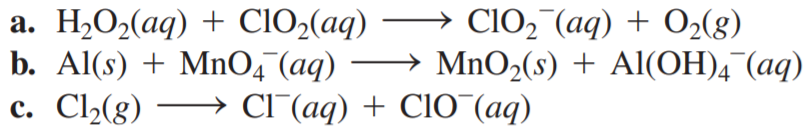×
Get Full Access to Chemistry: A Molecular Approach - 3 Edition - Chapter 18 - Problem 41e
Get Full Access to Chemistry: A Molecular Approach - 3 Edition - Chapter 18 - Problem 41e

×

# Balance each redox reaction occurring in basic aqueousISBN: 9780321809247 1

## Solution for problem 41E Chapter 18

Chemistry: A Molecular Approach | 3rd Edition

• Textbook Solutions
• 2901 Step-by-step solutions solved by professors and subject experts
• Get 24/7 help from StudySoup virtual teaching assistantsChemistry: A Molecular Approach | 3rd Edition

4 5 1 260 Reviews
26
1
Problem 41E

Balance each redox reaction occurring in basic aqueous solution.Step-by-Step Solution:

Problem 41E

Balance each redox reaction occurring in a basic aqueous solution.

a)b)c)Step by step solution

Step 1 of 3

a.

The equation for the unbalanced redox reaction is as follows:Step 1: Separate the overall reaction into two half-reactions: One for oxidation and one for reduction.Step 2 of 4

Step 3 of 4

## Discover and learn what students are asking

Statistics: Informed Decisions Using Data : Estimating a Population Standard Deviation
?True or False: A confidence interval for a population standard deviation is of the form point estimate ± margin of error.

Unlock Textbook Solution